PHY294H - Lecture 29

Nuclear magnetic resonance(NMR)

Nuclear magnetic resonance works by controlling the magnetic moment of nuclei. Usually a nucleus which has a net spin of 1/2 is used as this is the case where the theoretical analysis is well understood. The magnetic moment of a proton or a neutron is about 2000 times smaller than the magnetic moment of an electron. However if a large magnetic field is applied to a nucleus, the magnetic field produces a significant splitting of the up and down energy levels of the nuclear spin,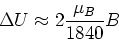(1)

where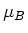is the Bohr magneton. Now an oscillatory field is applied and resonance occurs when,(2)

By measuring the resonant absorption, it is possible to figure out how many nuclear magnetic moments are present in the material. A closer loop at which is happening at the atomic level is evident upon considering a quantum mechanical magnetic dipole in the applied field B. The torque on the dipole is,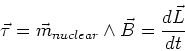(3)

The fundamental postulate of quantum mechanics is the the magnitude of L is fixed. This means that the quantum magnetic moment cannot align with the applied field. Instead it precesses around the direction of the magnetic field. The frequency of this precession is called the Larmour frequency. resonance occurs when the Larmour frequency is equal to the frequency of the applied oscillatory magnetic field.

In medical applications NMR is called MRI (medical resonance imaging) and the Hydrogen nucleus is used as the target nucleus (different nuclei have different resonant frequencies). MRI measures the local amount of absorption and so gives an estimate of the number of H nuclei in the part of the human body to which it is applied. Since fat has a high concentration of H nuclei, while bone has a low concentration, there is good contrast between fat and bone. MRI is complementary to X-rays which scatter most strongly from high mass nuclei. MRI can be extended to provide a probe of the velocity of the protons and also the local environment of the protons. This is call functional MRI.

Self-Inductance (L)

We now look at the way in which magnetic field effects are used in circuit applications. In circuit applications, we want to related the emf across a circuit element to the current through the element. Consider placing a solenoid in a circuit. We know that the magnetic field in the solenoid is given by,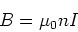(4)

where I is the current through the solenoid. The flux through the solenoid is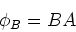(5)

where A is the cross-sectional area of the solenoid. The emf across the solenoid is then,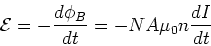(6)

where N = nl is the number of loops in the solenoid. It is thus evident that the induced emf across the solenoid is proportional to the rate of change of the current and that the induced emf opposes the change in current. This is the circuit version of Faraday's law. In circuits, we write,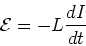(7)

where L is the inductance. This is true for any configuration of current carrying wires, not just for the solenoid. The only thing that depends on the wire geometry is the inductance. For a solenoid, we find,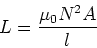(8)

The self-inductance thus tells us how the current and voltage are related in a current carrying wire configuration.

Mutual inductance

Since the magnetic fields of one current carring wire configuraiton may influence a second wire, it is natural to consider the way in which two wire configurations are coupled through their magnetic fields. This coupling is described by the mutual inductance. We write,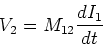(9)

Consider for example a small solenoid, of area A2 and N2 turns and of length l2 inside a solenoid (with N1 turns, length l2 and area A2) which carries current I1, so that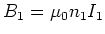. The flux passing through the small solenoid is. If we change the current I1, we change the flux through the loop so that,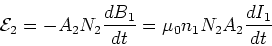(10)

The mutual inductance is then,(11)

Doing a similar calculation for the inner solenoid gives

 M21 = M12 = M (12)

Energy stored in inductors

The rate at which work is done in an inductor is given by,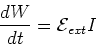(13)

We have shown that the voltage across an inductor is -LdI/dt, so we have,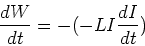(14)

The energy stored in an inductor is thus,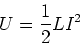(15)

This energy is stored in the magnetic field of the inductor, for example in a solenoid we have,(16)

The energy density in the magnetic field is then,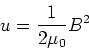(17)